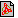Cím: Preparatory problems for the entrance exam of high school Szerző(k): László Számadó Füzet: 2002/decemberi melléklet, 45. oldalPDF file Témakör(ök): Felvételi előkészítő feladatsor

A szöveg csak Firefox böngészőben jelenik meg helyesen. Használja a fenti PDF file-ra mutató link-et a letöltésre.

1. Solve the following equation on the set of real numbers:

 $\begin{array}{c}\frac{2x+2}{7}=\frac{\left({x}^{2}-x-6\right)\left(x+1\right)}{{x}^{2}+2x-3}.\end{array}$

2. For what positive integers $a$ is the value of the following expression also an integer?
 $\begin{array}{c}\left(\frac{a+1}{1-a}+\frac{a-1}{a+1}-\frac{4{a}^{2}}{{a}^{2}-1}\right):\left(\frac{2}{{a}^{3}+{a}^{2}}-\frac{2-2a+2{a}^{2}}{{a}^{2}}\right)\end{array}$

3. Given that the second coordinates of the points $A\left(1,a\right)$, $B\left(3,b\right)$, $C\left(4,c\right)$ are
 $\begin{array}{c}a=-\frac{\text{sin}{39}^{\circ }+\text{sin}{13}^{\circ }}{\text{sin}{26}^{\circ }\cdot \text{cos}{13}^{\circ }},\phantom{\rule{2em}{0ex}}b=\sqrt[]{{10}^{2+\text{log}{}_{10}25}},\phantom{\rule{2em}{0ex}}c={\left(\frac{1}{\sqrt[]{5}-2}\right)}^{3}-{\left(\frac{1}{\sqrt[]{5}+2}\right)}^{3}\end{array}$
determine whether the three points are collinear.

4. What is more favourable:
I. If the bank pays $20%$ annual interest, and the inflation rate is $15%$ per year, or
II. if the bank pays $12%$ annual interest, and the inflation rate is $7%$ per year?

5. The first four terms of an arithmetic progression of integers are ${a}_{1},{a}_{2},{a}_{3},{a}_{4}$. Show that $1\cdot {a}_{1}^{2}+2\cdot {a}_{2}^{2}+3\cdot {a}_{3}^{2}+4\cdot {a}_{4}^{2}$ can be expressed as the sum of two perfect squares.

6. In an acute triangle $ABC$, the circle of diameter $AC$ intersects the line of the altitude from $B$ at the points $D$ and $E$, and the circle of diameter $AB$ intersects the line of the altitude from $C$ at the points $F$ and $G$. Show that the points $D$, $E$, $F$, $G$ lie on a circle.

7. The base of a right pyramid is a triangle $ABC$, the lengths of the sides are $AB=21\phantom{\rule{0.5ex}{0ex}}\text{cm}$, $BC=20\phantom{\rule{0.5ex}{0ex}}\text{cm}$ and $CA=13\phantom{\rule{0.5ex}{0ex}}\text{cm}$. $A\text{'}$, $B\text{'}$, $C\text{'}$ are points on the corresponding lateral edges, such that $AA\text{'}=5\phantom{\rule{0.5ex}{0ex}}\text{cm}$, $BB\text{'}=25\phantom{\rule{0.5ex}{0ex}}\text{cm}$ and $CC\text{'}=4\phantom{\rule{0.5ex}{0ex}}\text{cm}$. Find the angle of the planes of triangle $A\text{'}B\text{'}C\text{'}$ and triangle $ABC$.

8. Let $f\left(x\right)=2{x}^{6}-3{x}^{4}+{x}^{2}$. Prove that $f\left(\text{sin}\alpha \right)+f\left(\text{cos}\alpha \right)=0$.